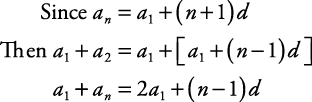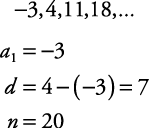## Arithmetic Series

An arithmetic series is the sum of the terms in an arithmetic sequence with a definite number of terms. Following is a simple formula for finding the sum:

Formula 1:

If S n represents the sum of an arithmetic sequence with terms, thenThis formula requires the values of the first and last terms and the number of terms.Substituting this last expression for ( a 1 + a n ) into Formula 1, another formula for the sum of an arithmetic sequence is formed.

Formula 2:This formula for the sum of an arithmetic sequence requires the first term, the common difference, and the number of terms.

##### Example 1

In the arithmetic sequence –3, 4, 11, 18, …, find the sum of the first 20 terms.Use Formula 2 to find the sum.##### Example 2

Find the sum of the multiples of 3 between 28 and 112.

The first multiple of 3 between 28 and 112 is 30, and the last multiple of 3 between 28 and 112 is 111. In order to use Formula 1, the number of terms must be known. a n = a 1 + ( n – 1) d can be used to find n.Now, use Formula 1.The sum of the multiples of 3 between 28 and 112 is 1974.

Back to Top
A18ACD436D5A3997E3DA2573E3FD792A